﻿ 基于高斯过程回归的船舶动力学模型辨识
 舰船科学技术2022, Vol. 44Issue (19): 1-5    DOI: 10.3404/j.issn.1672-7649.2022.19.001PDF

Identification of ship dynamics model based on Gaussian process regression
CHEN Gang, WANG Wei, HUO Cong
College of Naval Architecture and Ocean Engineering, Naval University of Engineering, Wuhan 430033, China
Abstract: Ship dynamics model is crucial for intelligent navigation and design of the ship’s controller, nonparametric regression based on Gaussian process regression is used for ship dynamics model identification. it can capture the strong nonlinearity and motion cou-pling in ship motion, and deal with the presence of uncertainty and noise. The identified model can provide ship acceleration, speed and position information for a period of time in the future when the sensor signal is lost. The experimental data of KVLCC2 ship are used to verify the validity of the proposed method, the results show that Gaussian process regression can provide accurate predictions of ship’s state, the position prediction error of 1000 steps is 0.599 m.
Key words: gaussian processes regression     identification     ship dynamics
0 引　言

1 船舶动力学参数模型和非参数模型

 $M\dot{s}+C\left(s\right)s+D\left(s\right)s+g\left(\eta \right)+{g}_{0}=\tau +{\tau }_{wind}+{\tau }_{wave}。$ (1)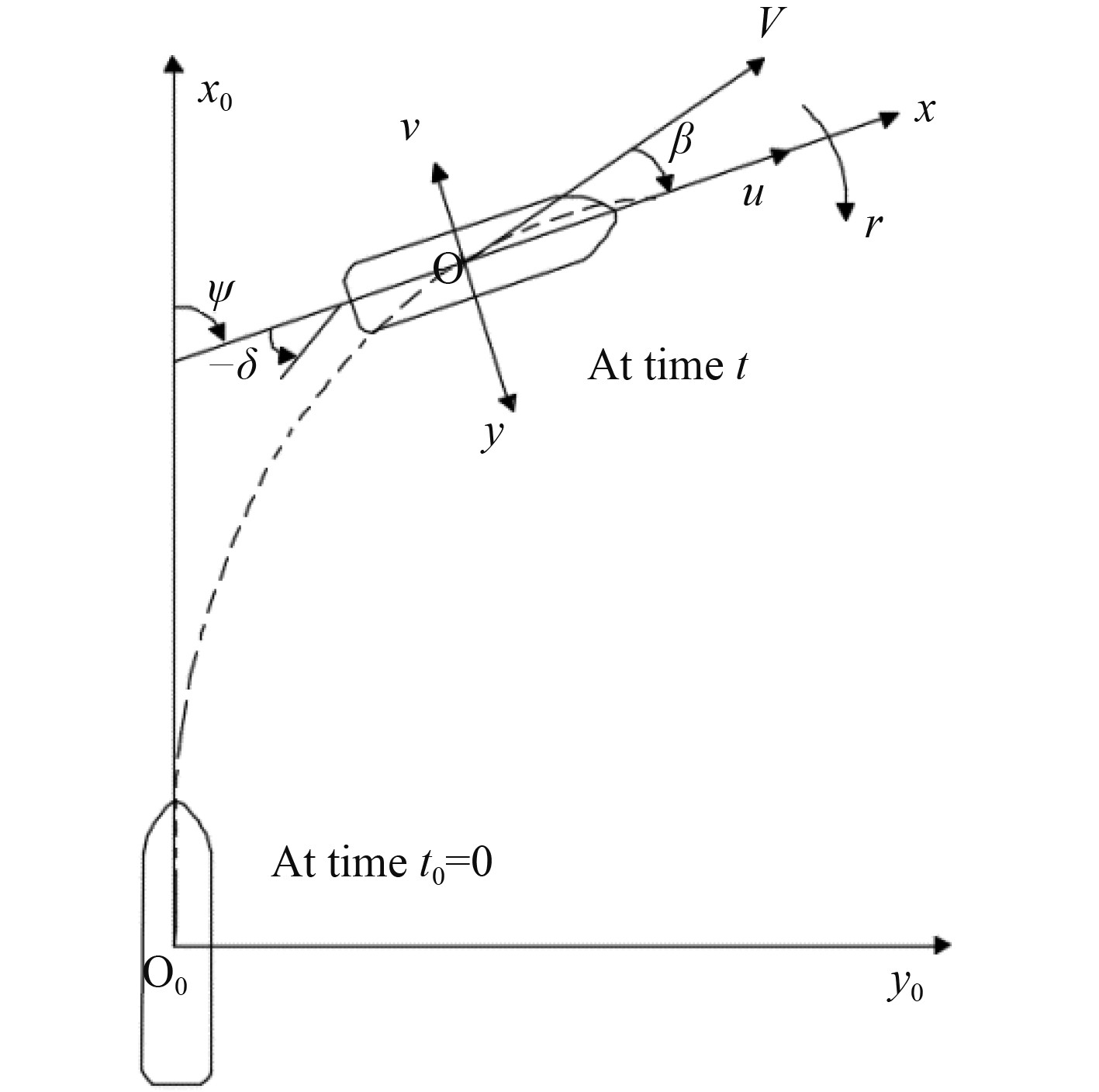图 1 地球和船舶固定坐标系 Fig. 1 Earth and ship-fixed coordinate systems

 $M\dot{s}+C\left(s\right)s+D\left(s\right)s=\tau。$ (2)

 $\dot{s}=f(s,\tau )。$ (3)

 ${\dot{u}}_{k}={f}_{u}\left({u}_{k},{v}_{k},{r}_{k},{T}_{k},{\delta }_{k}\right) ，$ (4)
 ${\dot{v}}_{k}={f}_{v}\left({u}_{k},{v}_{k},{r}_{k},{T}_{k},{\delta }_{k}\right)，$ (5)
 ${\dot{r}}_{k}={f}_{r}\left({u}_{k},{v}_{k},{r}_{k},{T}_{k},{\delta }_{k}\right) 。$ (6)
2 基于高斯过程回归的非参数建模 2.1 高斯过程回归

$\left\{({x}_{i},{y}_{i})|i=1,\cdots ,n\right\}$ 是回归的输入和输出，它们之间的关系可以表示为：

 ${y}_{i}=f\left({x}_{i}\right)+\varepsilon ,\varepsilon \sim N(0,{\mathrm{\sigma }}_{n}^{2})，$ (7)

 $\left[\begin{array}{c}y\\ {f(x}^{*})\end{array}\right] \sim N\left(0,\left[\begin{array}{cc}K\left(\mathrm{X},\mathrm{X}\right)+{{\mathrm{\sigma }}_{n}}^{2}I& K\left({x}^{*},\mathrm{X}\right)\\ K\left(\mathrm{X},{x}^{*}\right)& K\left({x}^{*},{x}^{*}\right)\end{array}\right]\right)，$ (8)

${f(x}^{*})$ 的预测值为：

 ${f(x}^{*})=k\left({x}^{*},X\right){\left(\mathrm{K}\right(\mathrm{X},\mathrm{X})+{{\mathrm{\sigma }}_{n}}^{2}{I}_{n})}^{-1}y ，$ (9)
 $\begin{split} Var\left[{f(x}^{*})\right]=&k\left({x}^{*},{x}^{*}\right)-\\ &k\left({x}^{*},X\right){\left(\mathrm{K}\right(\mathrm{X},\mathrm{X})+{{\mathrm{\sigma }}_{n}}^{2}{I}_{n})}^{-1}k\left(X,{x}^{*}\right) \end{split}，$ (10)

 $k\left({x}_{i},{x}_{j}\right)={f\left({x}_{i}\right)}^{{\rm{T}}}f\left({x}_{j}\right)={{\mathrm{\sigma }}_{f}}^{2}\mathrm{e}\mathrm{x}\mathrm{p}\left(-\frac{1}{2}({x}_{i}-{x}_{j})^{{\rm{T}}}\mathrm{\Lambda }({x}_{i}-{x}_{j})\right)。$ (11)

2.2 船舶运动状态多步预测模型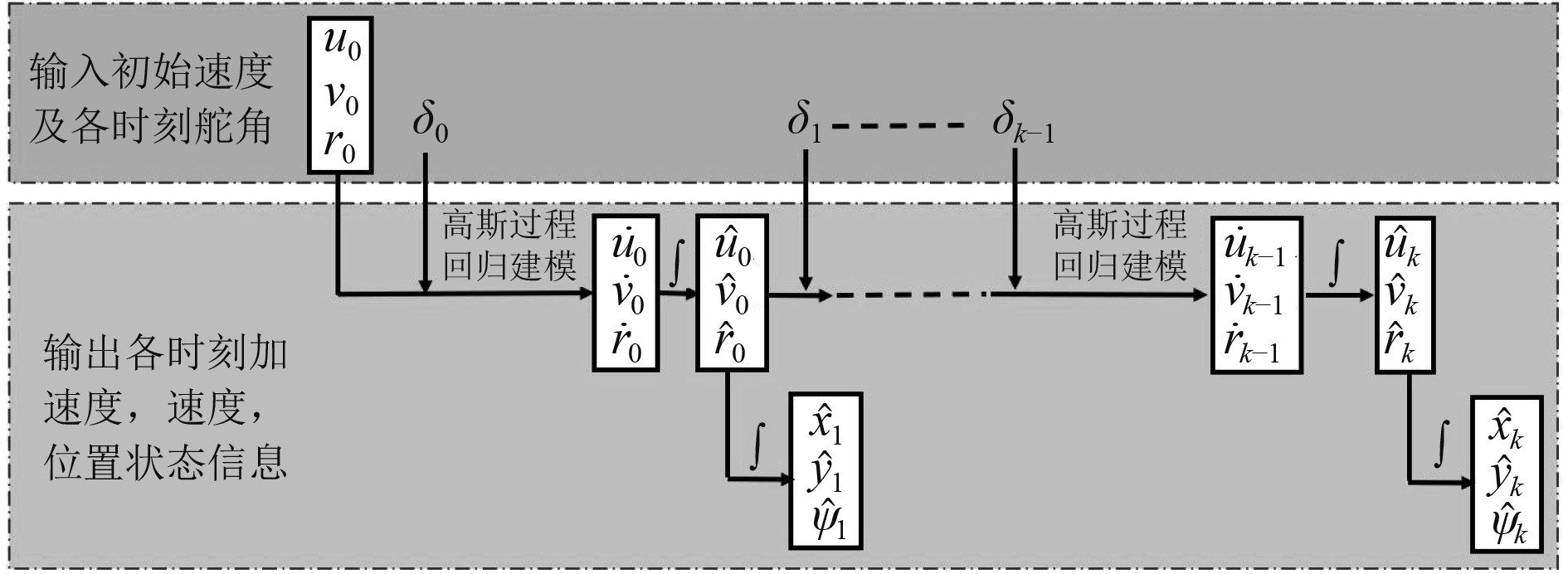图 2 多步预测模型的输入和输出 Fig. 2 The inputs and outputs of multi-step prediction model

3 KVCLL2船舶动力学辨识 3.1 实验船模型和数据集表 1 KVLCC2型号参数及尺寸 Tab.1 Parameters and dimensions of the KVLCC2 model表 2 KVLCC2试验数据 Tab.2 Test data of the KVLCC2 model
3.2 基于高斯过程回归的船舶动力学辨识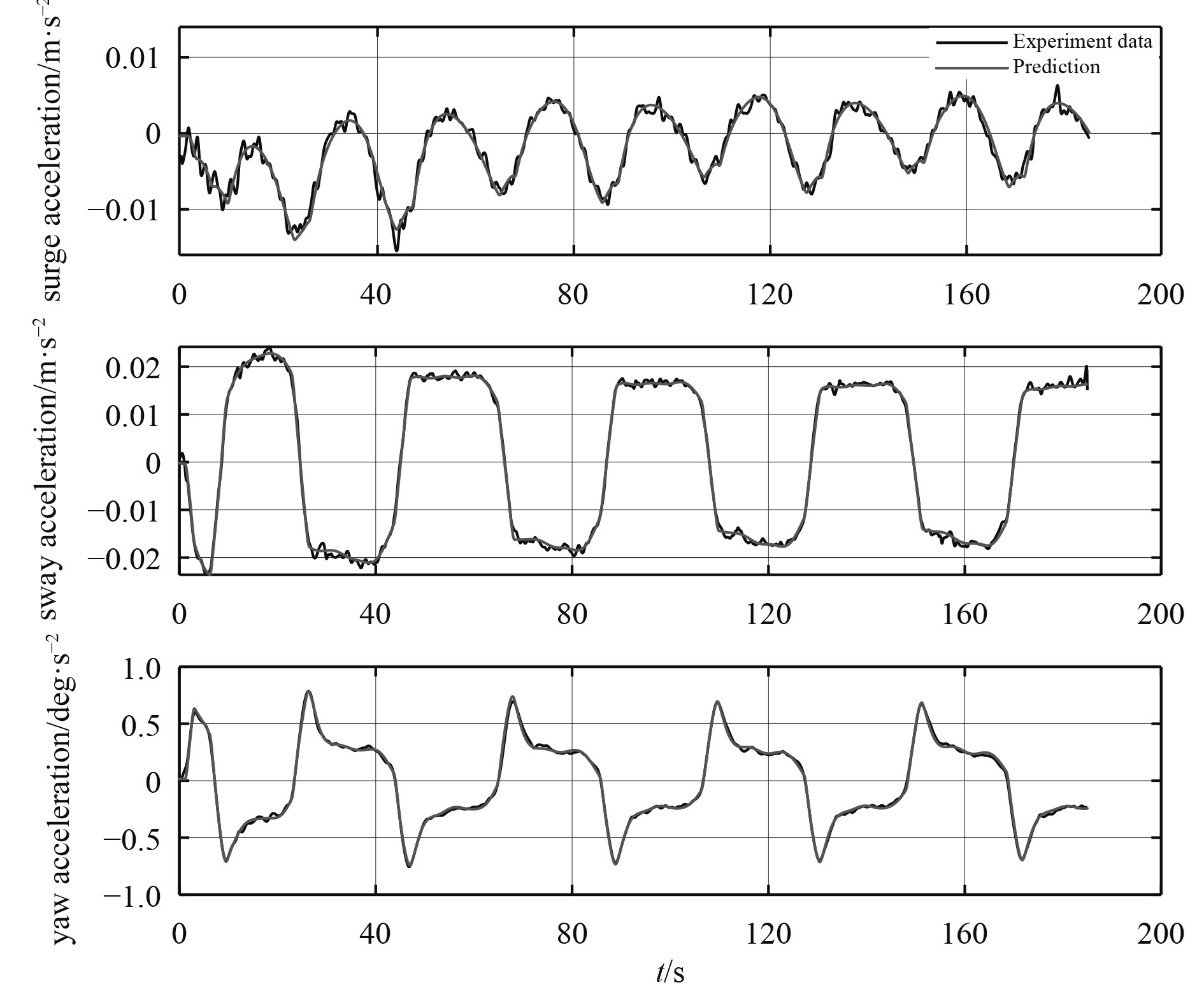图 3 25°/5°Z形运动中的加速度3700步预测 Fig. 3 3700 steps prediction of acceleration in 25°/5° zigzag maneuver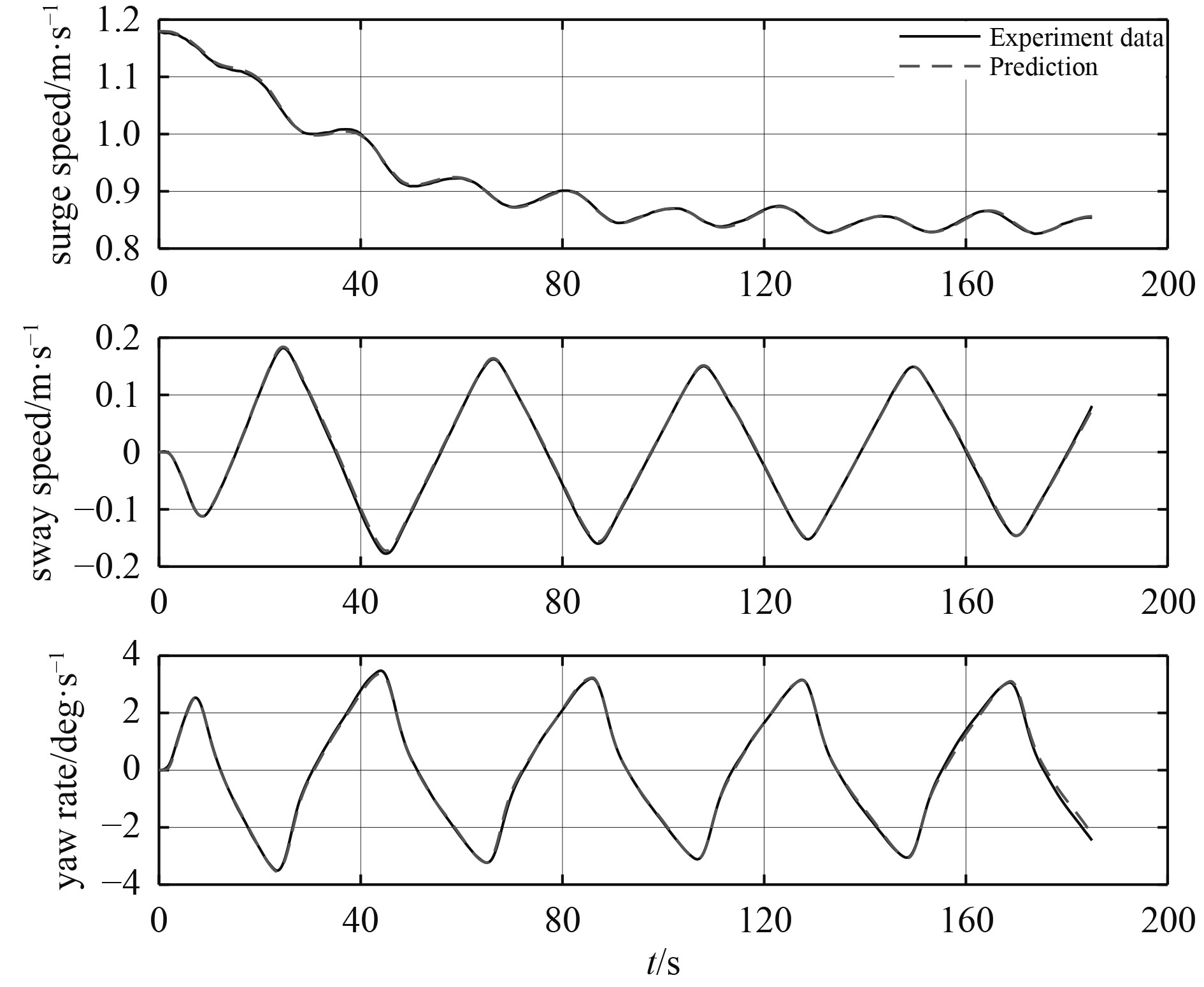图 4 25°/5°Z形运动中的速度3700步预测 Fig. 4 3700 steps prediction of speed in 25°/5° zigzag maneuver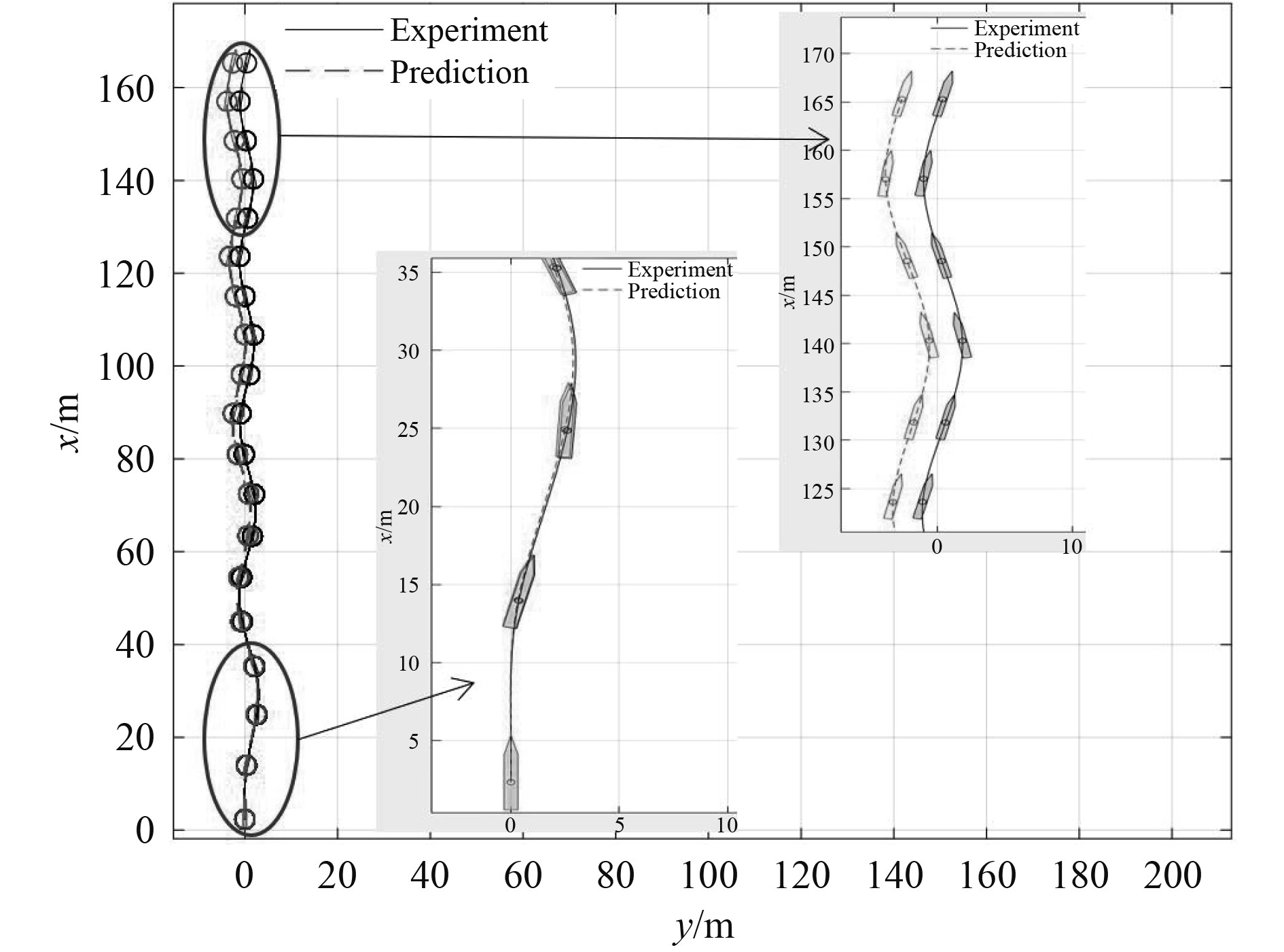图 5 25°/5°Z形运动中的轨迹3700步预测 Fig. 5 3700 steps prediction of position in 25°/5° zigzag maneuver表 3 25°/5°Z形试验多步预测RMSE的精度 Tab.3 Multi-step prediction accuracy assessed by the RMSE of the 25°/5° zigzag test

4 结　语

  ABKOWITZ M A. Measurements of hydrodynamic characteristics from ship maneuvering trials by system identification[C]// Transactions SNAME 88, 1980: 283−318.  NORRBIN N H. Ship Manoeuvring with Application to Shipborne Predictors and Real-Time Simulators[J]. Journal of Mechanical Engineering Science, 1972, 14(1): 91−107.  HOLZHUTER T. Robust identification scheme in an adaptive track-controller for ships[C]// IFAC Proceedings Volumes, 1990, 23: 461−466.  YOON H K, RHEE K P. Identification of hydrodynamic coefficients in ship maneuvering equations of motion by Estimation-Before-Modeling technique[J]. Ocean Engineering, 2003, 30(18): 2379−2404.  LUO W L, MOREIRA L, SOARES C G. Manoeuvring simulation of catamaran by using implicit models based on support vector machines[J]. Ocean Engineering, 2014, 82(15): 150−159.  ZHU M, HAHN A, WEN Y Q. Identification-based simplified model of large container ships using support vector machines and artificial bee colony algorithm[J]. Applied Ocean Research, 2017, 68(1): 249-261.  LUO W L, LI X. Measures to diminish the parameter drift in the modeling of ship manoeuvring using system identification[J]. Applied Ocean Research, 2017, 67(1): 9-20.  XU Haitong, HASSANI V, SOARES C G. Parameters Estimation of Nonlinear Manoeuvring Model for Marine Surface Ship Based on PMM Tests[C]. ASME 2018 37th International Conference on Ocean, Offshore and Arctic Engineering, 2018, 11: 19−23.  XU Haitong, SOARES C G. Experimental investigation of shallow water effect on vessel steering model using system identification method[J]. Ocean Engineering, 2017, 199: 106940.  XU Haitong, HASSANI V, SOARES C G. Uncertainty analysis of the hydrodynamic coefficients estimation of a nonlinear manoeuvring model based on planar motion mechanism tests[J]. Ocean Engineering, 2019, 173: 450−459.  XU H, HINOSTROZA M, HASSANI V, et al. Real-time parameter estimation of nonlinear vessel steering model using support vector machine[J]. Journal of Offshore Mechanics and Arctic Engineering, 2019, 141(6): 1−9.  MU D, WANG G, FAN Y, et al. Modeling and Identification for Vector Propulsion of an Unmanned Surface Vehicle: Three Degrees of Freedom Model and Response Model[J]. Sensors, 2018, 18(6): 1889.  SUTULO S, SOARES C G. An algorithm for offline identification of ship manoeuvring mathematical models from free-running tests[J]. Ocean Engineering, 2014, 15(79): 10−25.  WANG N, ER M J, HAN M. Large tanker motion model identification using generalized ellipsoidal basis function-based fuzzy neural networks[J]. IEEE Transactions on Cybernetics, 2015, 45(12): 2732−2743.  WANG Z, XU H, XIA L, et al. Kernel-based support vector regression for nonparametric modeling of ship maneuvering motion[J]. Ocean Engineering, 2014, 216: 10−25.  W, REN J, LI T. Modified genetic optimization-based locally weighted learning identification modeling of ship maneuvering with full scale trial [J]. Future Generation Computer Systems, 2019, 93: 1036−1045.  MORENO-SALINAS D, MORENO R, PEREIRA A, et al. Modelling of a surface marine vehicle with kernel ridge regression confidence machine[J]. Applied Soft Computing Journal, 2019, 76(1): 237−250.  MORENO R, MORENO-SALINAS D, Aranda J. Black-box marine vehicle identification with regression techniques for random manoeuvres[J]. Electronics, 2019, 8(5): 492−492.  MORENO R, BESADA-PORTAS E, ARANDA J, Symbolic regression for marine vehicles identification[J]. IFAC-Papers Online, 2015, 48(16): 210−216.  CARRON A, ARCARI E, WERMELINGER M, et al. Data-driven model predictive control for trajectory tracking with a robotic arm [J]. IEEE Robotics and Automation Letters, 2019, 4(4): 3578−3765.  KABZAN J, HEWING L, LINIGER A. Learning-based model predictive control for autonomous racing [J]. IEEE Robotics and Automation Letters, 2019, 4(4): 3363−3370.  RAMIRE W A, LEONG Z Q, HUNG N, et al. Non-parametric dynamic system identification of ships using multi-output Gaussian Processes[J]. Ocean Engineering, 2018, 166: 26−36.  XUE Y, LIU Y, JI C, et al. System identification of ship dynamic model based on Gaussian process regression with input noise [J]. Ocean Engineering, 2020, 216: 107862.  FOSSEN T I. Handbook of marine craft hydrodynamics and motion control[M]. 2011: 134−135.  RASMUSSEN C E, WILLIAMS K I. Gaussian processes for machine learning[M]. 2006: 8−31.